# Parameter inference for discretely observed stochastic kinetic models using stochastic gradient descent

## Abstract

### Background

Stochastic effects can be important for the behavior of processes involving small population numbers, so the study of stochastic models has become an important topic in the burgeoning field of computational systems biology. However analysis techniques for stochastic models have tended to lag behind their deterministic cousins due to the heavier computational demands of the statistical approaches for fitting the models to experimental data. There is a continuing need for more effective and efficient algorithms. In this article we focus on the parameter inference problem for stochastic kinetic models of biochemical reactions given discrete time-course observations of either some or all of the molecular species.

### Results

We propose an algorithm for inference of kinetic rate parameters based upon maximum likelihood using stochastic gradient descent (SGD). We derive a general formula for the gradient of the likelihood function given discrete time-course observations. The formula applies to any explicit functional form of the kinetic rate laws such as mass-action, Michaelis-Menten, etc. Our algorithm estimates the gradient of the likelihood function by reversible jump Markov chain Monte Carlo sampling (RJMCMC), and then gradient descent method is employed to obtain the maximum likelihood estimation of parameter values. Furthermore, we utilize flux balance analysis and show how to automatically construct reversible jump samplers for arbitrary biochemical reaction models. We provide RJMCMC sampling algorithms for both fully observed and partially observed time-course observation data. Our methods are illustrated with two examples: a birth-death model and an auto-regulatory gene network. We find good agreement of the inferred parameters with the actual parameters in both models.

### Conclusions

The SGD method proposed in the paper presents a general framework of inferring parameters for stochastic kinetic models. The method is computationally efficient and is effective for both partially and fully observed systems. Automatic construction of reversible jump samplers and general formulation of the likelihood gradient function makes our method applicable to a wide range of stochastic models. Furthermore our derivations can be useful for other purposes such as using the gradient information for parametric sensitivity analysis or using the reversible jump samplers for full Bayesian inference. The software implementing the algorithms is publicly available at http://cbcl.ics.uci.edu/sgd

## Background

It is becoming increasingly apparent that stochasticity, whether intrinsic or extrinsic, plays an important role in the dynamics and behavior of biological systems. In systems biology and the study of gene expression , the consequences of stochasticity can manifest in numerous ways such as slow promoter kinetics leading to gene transcription bursting [4, 5], finite-number effects and mRNA translation bursting , propagation of noise in gene regulatory cascades [4, 10], and phenotypic switching [11, 12]. In some cases, biological systems evolve to minimize the effects of noise such as through negative feedback loops , but there is also evidence that biology exploits randomness such as to create phenotypic diversity in populations thus allowing better adaptation to changing environments . With the growing awareness of stochasticity in biology and the increasing use of stochastic models in computational systems biology, there is a need to develop new analysis and computational techniques for studying, understanding and designing these stochastic models.

One particular analysis technique and challenge in computational systems biology is the inference of rate parameters from experimental data for a specified biochemical system . Parameter inference for continuous deterministic models has a considerable body of research literature and can often be converted into an optimization problem for which many computational methods are available . The strategies of these methods can be classified as either deterministic or stochastic. Deterministic strategies are generally only applicable for specific mathematical formulations of the model where a statement about the existence of the global optimum can be guaranteed along with a constructive algorithm to find it. Many problems are not that well-defined so stochastic strategies are popular including stochastic gradient descent , simulated annealing , evolutionary computation , and other heuristics. Regardless, considerable computational effort is required for all of these methods as many simulations of the continuous deterministic model are performed. A discrete stochastic model is essentially a more adequate description for a biochemical system, but it has the disadvantage of being computationally expensive to simulate as well as requiring numerous independent simulations to be performed in order to calculate expectation values of various model outputs . These computational challenges mean that approximation techniques are frequently used for parameter inference including simplification of the stochastic model  and approximate inference such as using the chemical Langevin equation  in place of the Markov jump process [31, 32]. Recent research has shown that parameter inference for stochastic models is feasible given time course observations of the system, even if only a partial set of molecular species are observed [32, 33]. However the current algorithms, based on the Bayesian framework, are typically time-consuming due to the need of sampling high dimensional space. Therefore there are significant challenges in applying the method to real systems, such as gene regulatory networks .

Most proposed methods for parameter inference in stochastic biochemical models consider how to calculate the maximum likelihood for the rate parameter values given a stochastic model and observational data. Except for the simple models, the likelihood function is computationally intractable, so these methods either perform exact inference on an approximated model where the likelihood computation is tractable, or they approximate the likelihood with a more tractable function, or some combination of the two. Tian et al.  considered the simulated maximum likelihood (SML) method that estimates likelihood by generating samples from many simulations of the stochastic model. The ratio of samples matching observations to the total number of samples is used to estimate the transitional density and the log-likelihood. Then a genetic algorithm is used to obtain the optimal rate parameter values that minimize the log-likelihood function. While the SML approach is straightforward, it is computationally expensive because it requires a large number of simulations of the stochastic model. Similarly, approximate Bayesian computation also requires the stochastic model to be simulated, but it avoids calculating the likelihood function by comparing simulated data with observations using a rejection sampler [36, 37]. In a similar framework, Yosiphon et al.  used a simulated annealing procedure in an MCMC algorithm to estimate the parameters in stochastic models of reaction networks. Reinker et al.  proposed a method utilizing a hidden Markov model to approximate the stochastic model that takes observational error into account. Boys et al.  showed how full Bayesian inference can be performed on the stochastic Lotka-Volterra model along with performance of various Markov chain Monte Carlo (MCMC) algorithms. Interestingly they showed that with partially observed data, i.e., only one of the two species in the model, they can still make inferences about all three rate parameters in the model; though it is unclear how well this would work on larger models with many parameters. Wilkinson and colleagues have investigated additional methods including using diffusion approximations [29, 31] and incorporating multiple data sources .

In this paper, we describe an alternative method for parameter inference in discretely observed stochastic kinetic models. Instead of calculating and approximating the likelihood function as in the previous methods, we focus on estimating the gradients of the likelihood function with respect to the parameters. In particular, we propose a general methodology for efficiently estimating the gradients using reversible jump Markov chain Monte Carlo (RJMCMC). RJMCMC is an extension of the standard MCMC method that allows for generating samples on spaces of varying dimensions . An implementation challenge for RJMCMC is the lack of a general way to construct the jump proposals such that detailed balance is preserved . For stochastic kinetic models, the jump proposal corresponds to moves that change the number and the time of reactions that occur between two observations of the system. For most models, there is an infinite set of possible reaction processes (constrained by the observation data) that can occur between two time points, and the probabilities of different reaction paths depend upon the rate parameter values. Utilizing the research in flux balance analysis for metabolic networks , we provide an algorithm so that jump proposals can be automatically constructed from any standard biochemical model, thus allowing RJMCMC to be used without requiring any manual analysis by the modeler.

The availability of the gradient information allows for inference of the rate parameters of stochastic kinetic models using gradient descent-based methods. We implement a steepest gradient descent method for parameter inference using the estimated gradient information in a MATLAB software package http://cbcl.ics.uci.edu/sgd. We demonstrate the utility of our algorithms using two example stochastic models, including a birth-death process and a gene auto-regulation model.

## Methods

### Stochastic kinetic model of reaction systems with discrete states

Consider a general reaction system involving M reactions R 1 , R 2 ,..., R M and K species S 1 , S 2 ,..., S K . We denote the state of the reaction system by X = (x1,,x K ) where x a is the number of species S a . Each reaction Ri has an associated rate law, represented by a hazard function h i (X, Θ) (also called rate function), where Θ ≡ {θ r } is a set of parameters associated with the reactions. Suppose the reaction system takes the following form(1.1)

where u ra and v ra are the positive integer stoichiometries associated with reaction R r for reactant S a , representing the amount of species S a that decrease and increase respectively when reaction R r occurs. Eq. (1.1) can be represented more compactly as USVS, where U = [u ij ] and V = [v ij ] are M × K matrices. We define the net effect reaction matrix A = V - U, which reflects the net change of species numbers associated with reactions.

Asumming the reaction system is in well-stirred condition with a fixed volume, we can introduce the master equation model, also known as "chemical master equation (CME)" in the biochemical modeling field , which describe the time evolution of the state probability using a set of ordinary differential equations. The CME can be derived for any biochemical reaction system using the standard continuous time Markov process theory. Denote P(X;t) the probability of the system in state X at time t. For an infinitesimal time increment Δt, P(X;t + Δt) can be written as the sum of probabilities of the number of ways in which the system can reach or leave the current state: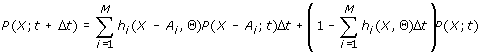(1.2)

where A i denotes the i th row of the net effect matrix A, and h i (X, Θ) is the hazard function, determining the rate of probability transition out of state X due to reaction type i. In the limit of Δt → 0, Eq. (1.2) adopts the standard master equation form,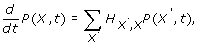(1.3)

with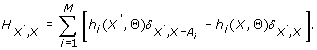(1.4)

Suppose all possible system states (usually countably infinite) are ordered and represented by indices 1, 2,..., etc. Then Eq. (1.3) can be rewritten as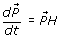, where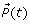is a row vector with P i (t) representing the probability of the i-th state at time t.

For reactions that obey mass-action law kinetics, one rate parameter θ r is associated with each reaction type i, and consequently the hazard function has the form of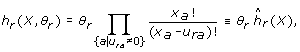(1.5)

where u ra is the stoichiometry coefficient of reactants a in reaction R r . Forms of other rate laws for chemical kinetics, e.g. the Michaelis-Menten model, can be found in . Although we will focus our discussion on the hazard function in the form of Eq.(1.5), the following analysis can handle more general cases as long as the explicit functional form of the hazard function is known.

### Gradient of the likelihood function with discrete observations

Our goal is to estimate the rate parameters of a stochastic model based on the observations at a set of discrete time points. Suppose we have observations {XΓ(t1), XΓ(t2),,XΓ(t m )} of the system at m discrete time points {t1, t2,...,t m } for a subset of species Γ {1,,K}. We say the system is fully observed if Γ = {1,,K}, and partially observed otherwise. Denoting the likelihood of the observations for a given set of rate parameters by L(XΓ(t1), XΓ(t2),,XΓ(t m ); Θ), we estimate the rate parameters by maximizing the likelihood function.

For simplicity of discussion, consider first a single time interval [ts, ts+1] with full observations available at the start and the end of the interval, denoted by X(ts) and X(ts+1) respectively. Let L(X(t s ), X(ts+1);Θ) denote the likelihood of observing X(ts) and X(ts+1) under a model with parameters Θ. In Appendix, we show that the gradient of the likelihood function with respective to parameters can be calculated using the following formula, for any stochastic system with a master equation Eq. (1.3)(2.1)

where Tk is the time duration of the system at state k, and N k, k' is the number of transitions from state k to k' occurred during the interval. Both Tk and N k, k' are random variables, and can be viewed as the sufficient statistics of the model. E[·] represents the expectation of the random variables. The formula suggests that we can calculate the gradient of the likelihood function by estimating the expectations of the two sufficient statistics.

For the biochemical reaction system in Eq. (1.1), suppose J reactions have occurred during the time interval [t s , ts+1] with the types and the corresponding times of the reactions denoted by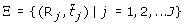. Then by Eq. (2.1), the gradient of the likelihood function can be rewritten as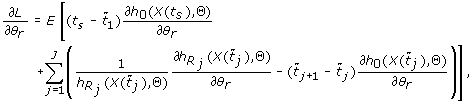(2.2)

where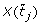, which is fully specified by Ξ, denotes the state of the system between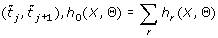, and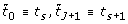. Eq. (2.2) can also be written in an alternative form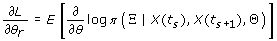(2.3)

where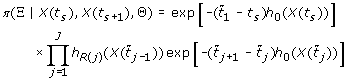is the likelihood of the reaction process Ξ. If all the reactions follow mass-action law in Eq.(1.5), the gradient formula can be further written as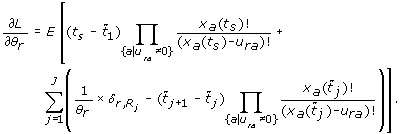(2.4)

Now return to the general case where the observations are available at multiple time points from a subset of the species. The above formula for calculating gradient can still hold if we view the entire duration of the observations as a single time interval. However, the expectation in Eq. (2.3) is now taken on the systems states whose distribution is conditioned on the observations at the intermediate time points.

In general, the expectation in Eq. (2.3) cannot be calculated exactly. Instead we utilize a sampling method to approximate the expectation. More specifically, we sample the latent path conditioned on the parameters and the observations, and then calculate the quantity in Eq. (2.3) by averaging over the sampled paths to obtain the gradient. The same strategy also applies to the partially observed case, as long as the reaction paths are sampled conditioned on the partial observation data.

### Reversible jump Markov chain Monte Carlo sampling

To calculate the gradient, we need to find an efficient way to sample the latent reaction processes conditioned on the observations. One commonly used sampling method is the stochastic simulation algorithm (SSA) , which can be used as a rejection method to discard samples that do that match the end state. The SSA method is computationally inefficient for generating samples between two measurements when the total number of possible states is high (as in the case of the biochemical reactions), because the chance of a sampled trajectory matching the end state is typically small and consequently most of the samples will likely be rejected.

Here we use the framework of RJMCMC  to sample the latent process. RJMCMC is a generalized MCMC method that can construct a sampler between models of different dimensions, which in our case corresponds to reaction paths with different number of reactions. To sample latent paths in biochemical reaction systems, the RJMCMC method  first generates an initial reaction path that is consistent with the observations. Then RJMCMCM constructs a Markov chain by a) proposing a new sample path by adding or deleting a specific set of reactions from the current path, and b) determining whether to accept the new sample or keep the previous one according to an acceptance probability.

Therefore, to construct a RJMCMC sampler, we will need to consider three issues: 1) how to generate the initial path; 2) how to propose a set of reactions for addition or deletion; and 3) how to determine the acceptance probability of a new path. Note that both the initial path and the proposed path have to match the observations at the start and the end of the interval, implying that only a subset of the reactions can be used for either initialization or addition/deletion. While the RJMCMC sampler exists for some specific reaction systems , usually taking advantage of the domain-specific knowledge, the challenge, however, is to find a general method that can work for any arbitrary reaction system.

Next we address the three issues mentioned above, and describe a general method to automatically construct a RJMCMC sampler for an arbitrary reaction system.

#### 1) Generating an initial reaction path using integer programming

The first issue of generating the initial path is relatively easy to address. Let r be a vector representing the number of each reaction type occurred within the initial path. To match the observations at the start and the end of each interval, r has to satisfy certain constraints. Fortunately, all these constraints are linear, and thus we can use linear integer programming to find a solution. In practice, we used the GNU Linear Programming Kit (GLPK library) , which is incorporated into our MATLAB package using the interface GLPKMEX .

#### 2) Proposing a new sample by adding or removing reactions

After an initial path is generated, our next step is to use proposal moves to add or remove reactions. Before describing our method, we first introduce two concepts that are used in studying biochemical reaction systems.

#### Definition 1: Elementary Mode

An elementary mode (EM) of a biochemical reaction network is a set of reactions that does not alter the observed number of molecular species. Formally, an elementary modeis a column vector of non-negative integers that satisfies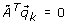, where Ã = A ( the net-effect reaction matrix) when all species are observable, and is a sub-matrix of A with columns corresponding to the observed species when only a subset of species are observable.

#### Definition 2: Null Set

The null set is a set consisting of all independent elementary modes, denoted by.

Note that the null set is usually different between the fully and the partially observed case because of the different Ã matrix used.

Elementary modes analysis is well studied in metabolic networks theory and is used to find the flux distribution of the metabolic network at a steady state . Various tools have been developed to identify EM s . In this work, we used the metatool package  to calculate the null set of any specific reaction network, which has been shown to be efficient for large networks.

Provided with a valid reaction path and the null set, we then proceed to generate a new sample by taking one of the following three move types. After randomly choosing an elementary mode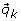from the null set,

1. 1.

With probability α1, add the set of reactions inwith random reaction times uniformly distributed within the interval.

2. 2.

With probability α2, remove one set of randomly selected reactions infrom the current path within the interval.

3. 3.

With probability 1-α12, randomly move the time of all reactions.

Usingensures that the proposed reaction path is always consistent with the observations. However, there are two additional conditions for a new sample path to be valid: 1) the number of any reaction type must be positive after the move, and 2) the population numbers for all species remain positive throughout the whole process. If either of the two conditions is violated, we set the likelihood of the new sample path to be zero and reject the new sampled path. The proposal probability in RJMCMC for different moves is set be to α1 = α2 = 0.25 in practice. Note that the initial path and the null set only need to be calculated once, and thus they only impose a modest computational burden on the sampling algorithm.

#### 3) Determining acceptance probability

Next we address the third issue on how to determine the acceptance probability of a proposed sample. We discuss the fully observed case first, and then the partially observed case.

#### Fully observed case

The observations at m discrete time points break the entire observation window into m-1 subintervals. Because all species are observed, the reaction path at each sub-interval is completely independently of each other conditioned on the observations. The reaction path at each sub-interval can therefore be sampled independently using RJMCMC. Let Ξ denote the current reaction path and Ξ denote the proposed reaction path. The probability of accepting the new path is specified by min(1, AR p ), with p = 1, 2, or 3 denoting the type of the movewhere π(Ξ|Θ), defined in Eq. (2.3), is the likelihood of sample path Ξ, rj is the number of type j reaction in the current sample path, q k, j denotes the number of reaction type j in the elementary mode, and τ is the time length of the sub-interval. Appendix, Algorithm 1 provides the pseudo-code for the fully observed case.

#### Partially observed case

In the partially observed case, observations are only available for a subset of the species. Different from the fully observed case, the reaction paths at different sub-intervals are now correlated, caused by unobserved species. Consequently, RJMCMC can no longer be applied independently for each sub-interval.

To account for the correlation, we use a new strategy in which the reaction paths at two consecutive sub-intervals are sampled together at each sampling step using correlated moves. Let {q k '}, k (1, K') be the null set corresponding to the partially observed case. Note that adding/deleting the set of reactions in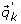only ensures that the observed species' numbers remain unchanged, but not the unobserved species. Suppose we are to update the reaction path following the time point t i . We first generate a new sample path in the i-th interval [t i , ti+1] using the same reversible jump moves as described for the fully observed case, with a randomly chosen elementary mode. If the move changes the unobserved species numbers at time ti+1, we subsequently update the (i+1)-th interval using a complementary move that keeps the system state at the end of the second interval unchanged. For example, if move type 1 (or 2) is chosen to update the first interval with an elementary mode q' k , move type 2 (or 1) will be applied to the second interval to remove (or add) the same elementary mode q' k . The complementary moves guarantee that the new reaction paths proposed for the two sub-intervals do not change the species numbers, including those of the unobserved species, at the end of the second interval. As with the fully observed case, the two conditions of a valid path (positive reaction type number and positive species number) must be satisfied, otherwise the proposal move will be rejected. The acceptance probability is calculated as, where p' denotes the complementary move type of p. In this way, the state of unobserved species at time t i (i = 2,,m) can be updated sequentially. An additional step is used to update the state at the first observation time point t1, which is done by keeping the species number at the end of the first interval fixed and changing the start state according to the proposed move. Appendix, Algorithm 2 provides the pseudo-code of using RJMCMC for the partially observed case.

Given the estimated gradient of the likelihood function, we use the method of steepest descent to find an optimal solution of the parameters. At each step of the algorithm, we first generate sample paths using the RJMCMC algorithm at current parameter values. After burn-in, we calculate the gradient of the likelihood function using the formula in (2.4). The estimated gradient is then used to update the parameter values until convergence. A simple strategy for choosing the step size is to set it to be a constant. Although this works well for simple systems, it sometimes induces over-shooting of the parameter values or slow convergence during the gradient descent. When this happens, we adaptively adjust the step size within a certain range according to the gradient value. An overview of the stochastic gradient descent algorithm is given in Appendix, Algorithm 3.

## Results

Next we illustrate the utility of our algorithm using two example reaction systems. In both cases, we simulated the reactions of the system using the stochastic simulation algorithm, and recorded the species numbers at a set of discrete time points, which were treated as observations of the system. Our method was then applied to infer the rate parameters for each system based on these observations.

### Example 1: Birth-death process

We first applied our algorithm to a well-studied birth-death process, which can be seen as a simplified model of production and degradation of a single molecular species . The reactions areWe assume that R1 and R2 follow the first-order and zeroth-order mass-action law respectively. Denote the number of A molecules by n A , thus the hazard function is given by h1 = k1n A and h2 = k2. The net-effect reaction matrix of the system is A = (-1, 1)T. Consequently, the null set of the system contains only one elementary mode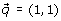, i.e. the combination of R1 and R2.

We generated observations by simulating the reaction process using SSA with different parameter sets (k1, k2) = (0.03,0.6), (0.06, 0.6), (0.1, 0.6), (0.1, 0.3) and (0.03, 0.2). For each parameter set, four observation datasets were generated that differ on the total observation time (T) and the observation interval (Δt) (see Table 1).

We first examined the convergence property of the RJMCMC sampler with the different datasets generated with the first parameter set. Figure 1 shows the trace plots and autocorrelations of the total number of reactions in the sample paths. In all cases, we found the RJMCMC sampler is efficient and induces good mixing of sample paths, with convergence occurring typically within 100 samples. As expected, larger correlation length is observed for data with longer observation intervals (Figure 1d and 1f).

We applied the SGD algorithm to estimate the two rate parameters for each dataset. The convergence criterion is set to be that the relative changes of all parameter values are less than 0.005. We used 1000 samples after a burn-in of 100 samples to estimate the gradient for a given set of parameter values. The estimated parameters for each dataset are summarized in Table 1. In all cases, the inferred parameters showed a good agreement with the true values, although the accuracy of the estimation clearly correlates with the number of observations and the observation time intervals. For datasets with larger observation interval and fewer data points, larger variation is the observed for the inferred value between different datasets, indicating the parameters are less constrained in these cases (results not shown). Additional file 1, Figure S1 shows a typical gradient descent run using the one of the datasets generated with (k1, k2) = (0.03,0.6), which consists of 21 data points with a total time period of T = 40. We observed that the parameters converge very quickly during the gradient descent, typically within 20 steps for our tested random start values.

### Example 2: Prokaryotic auto-regulatory gene network

The second model we tested is a prokaryotic auto-regulatory gene network in which dimmers of a protein repress its own gene transcription by binding to a regulatory region upstream of the gene. The system, involving both transcription and translation, can serve as a simple, yet illustrative, example of gene regulation [31, 39]. The reactions in the network are given below: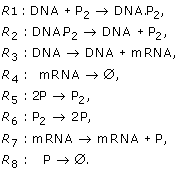Here DNA, P, P2 and mRNA represent promoter sequences, proteins, protein dimmers and messenger RNA respectively. In this model, mRNAs and proteins are synthesized by transcription and translation processes (R3 and R7), and destroyed by degradation (R4 and R8). The proteins can form a dimmer P2 (R5 and R6), which binds and unbinds to DNA (R1 and R2). When a protein dimmer binds to the promoter, it represses mRNA production. Overall, the network implements a self-regulatory mechanism to control the synthesis of the protein product, suppressing the transcription when the protein product is abundant. Note that DNA t = DNA + DNA.P2 is a conserved quantity in the system. The rate functions of reactions are assumed to follow mass-action law with rate parameters k1 to k8, e.g. h1 = k1·P2·DNA.

We applied our algorithm to both the fully and partially observed cases. We generated 10 datasets as observations within a time window of [0 50) with (k1,,k8) = (0.1, 0.7, 0.143, 0.35, 0.3, 0.1, 0.9, 0.11, 0.2, 0.1). Datasets D1 - D5 have total copy number DNA t to be 10 with the time interval between observations (Δt) from 1.0 to 0.1. The other five datasets D6 - D10 are generated with DNA t = 2. Detailed information of the datasets is shown in Table 2. For the partially observed case, we assume that only three of the species, mRNA, P and P2, are observed. In addition, we assume that the total copy number DNA t is known to avoid systematic bias in the sampling the system. While using the same datasets in the fully observed case, we only retain the observations corresponding to mRNA, P and P2. Hereinafter, we denote the datasets by D1*, D2* etc.

For the fully observed case, the net effect reaction matrix is, shown with the corresponding reactions and species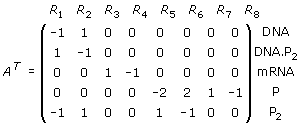The corresponding null set contains four elementary modes, consisting of the following four pairs of reactions: R 1 - R 2 , R 3 - R 4 , R 5 - R6, and R7 - R8.

We focus our analysis on datasets D1 - D5, of which the observation intervals range from 1.0 to 0.1. The results from datasets D6 - D10 are similar. The convergence property of the RJMCMC sampler is shown in Figure 2. It shows the RJMCMC sampler is efficient and induces good mixing for all the datasets, with convergence occurring typically after 200 samples. The correlation lengths between the samples are smaller for the more densely observed dataset (D5 with Δt = 0.1). The correlation length increases for the datasets with increasing Δt, suggesting the need of using larger sample size for sparse observed datasets.

We applied the stochastic gradient descent method to estimate the rate parameters given the observations. The initial parameter values were randomly chosen between 0.1 and 10. We used 5000 samples to calculate the gradient with a burn-in size of 200. The estimated parameters for each dataset are summarized in Table 2. We observed a good agreement between the estimated and true values for most of the parameters. Also we observed that there is differences in the estimation accuracy for different parameters, with some (e.g. k2, k3 and k4) showing consistently better results than others (Additional file 1, Figure S2). The estimation of k5 and k6 showed large deviation for the first two datasets with large observation interval (D1 and D2, Table 3), but improved with finer-sampled data. This is likely due to the faster dynamics of the two reactions (R5 and R6) than other reactions in the system.

Next we applied our algorithm to the partially observed case. The convergence property of the RJMCMC sampler for the partially observed case is shown in Figure 3. Compared with the fully observed case, the autocorrelation length in the partially observed case is typically longer, but the RJMCMC sampler can still induce good mixing for each dataset with adequately large sample size.

The parameter inference results are summarized in Table 3. We found that the accuracy of the inferred parameter varies for different datasets. For the densely observed dataset D5*, the estimated values of all eight parameters are similar to those in the fully observed case and close to the true values. But for more sparsely observed datasets, the average percent of error of the inferred parameters increases significantly (compared to the fully observed case) for some of the datasets (D1* and D4*). The parameters k1 and k2, which are associated with the unobserved species, showed large variations between different datasets. In general, the results showed that parameter inference with partially observed data is more difficult than the one with fully observed data, and to achieve good estimation accuracy, more observations with small observation intervals will be needed.

Additional file 1, Figure S3 shows the changes of parameters and gradients during one gradient descent run for the most sparsely observed dataset D1 and D1* with the dataset (copy number of each species) shown in Additional file 1, Figure S4. Some of the parameters (e.g. k2 and k6) showed slow convergence during gradient descent in both fully and partially observed cases, which may reflect a flat likelihood surface in the corresponding parameter direction and an inherent difficulty in identifying these parameters.

## Discussions

Recently there has been a growing interest in describing biological systems using stochastic models. However, most of the parameters in the stochastic models are unknown and difficult to measure. In this paper we described a maximum likelihood method to infer the parameters of a stochastic kinetic model directly from observations. Our method works by estimating the gradient of the likelihood function first, and then searching for an optimal solution by iteratively updating the parameters along the gradient descent direction. We developed a general RJMCMC algorithm to sample the latent reaction path in a constrained setting, where the reaction path has to match the observations given at the two ends of a time interval. The sampled reaction paths are used to calculate the gradient of the likelihood function using a formula that we derived. The availability of the gradient information makes it possible to develop other algorithms to solve the maximum likelihood estimation problem, in addition to the steepest descent method that we implemented. Furthermore, the availability of the gradient information also enables other possible applications such as parameter sensitivity analysis, which has already attracted considerable interest in deterministic modeling [50, 51].

Our method is significantly faster than the SML method , which is also a maximum likelihood based parameter inference method. SML uses two steps to estimate parameters. First, it estimates the transition density on reaction species numbers after a given time interval, using a SSA-based sampling methods. The estimated transition density is then used to calculate the likelihood function. Because the gradient of the likelihood function is not directly available, SML uses a genetic algorithm to solve the maximum likelihood problem. Comparing the SML and our method for the birth-death example, we tested the CPU time used to generate a new sample for both methods, eg. SSA for SML (unconstrained) and RJMCMC for SGD, which is approximately the same. However, SML uses 3 × 104 evaluations of transition density to reach a solution. By contrast, SGD typically requires less than 20 evaluations of the gradient before convergence. If we ignore the computational time of the gradient descent steps, overall SGD achieves a reduction of computational time by an order of 10 3 compared to SML.

In terms of accuracy, our approach, based on exact sampling, should be less biased than approximation-based methods. In this regard, we compared SGD to the method by Golightly et al. , who used a diffusion approximation to calculate the transition density. Comparing the results obtained by both methods on the same datasets (in courtesy of Dr. Golightly), we note that the estimated values for k1 and k2 by our method are closer to the true results in all three test datasets while the result from  are biased toward low values, although the estimates for other parameters from the two methods are similar (Additional file 1, Table S1). Interestingly, k1 and k2 are associated with low copy number of reaction species (DNA and DNA.P2). We also tested the method in  with the datasets of DNA t = 2 and found that the algorithm gives worse results, especially for the first two parameters (result not shown). This reflects the advantage of our method and possibly the limitation of the diffusion approximation, which assumes that the values of the hazard functions are approximately constants between two observation/latent states. This assumption is not valid if the copy numbers of species are small in the reactions. For example, in case of DNA t = 1, reactions R1 and R2 can only happen alternatively and this clearly violates the approximation assumption.

Our method is closely related to the full Bayesian approach proposed by Boys et al.  as both methods use RJMCMC to sample the reaction process. Comparing to the method by Boys et al., our method offers two improvements. First, we provide a general method for RCMCMC sampling, which can be applied to an arbitrary biochemical reaction system, while the previous method is only tailored to a specific reaction system (more specifically, the Lotka-Volterra system). Second, the gradient-based method is significantly faster than the full Bayesian method as sampling the parameter space is often computationally challenging. However, the Bayesian approach offers certain advantage over the maximum likelihood method in that it provides a posterior distribution of the parameters rather than just an optimal solution. In this regard, we note that the general RJMCMC sampling method we developed can be easily extended for Bayesian inference after introducing additional Metropolis-Hasting steps for sampling parameters.

## Conclusion

In this paper, we proposed a new algorithm for inferring rate parameters in stochastic models and tested it using simulated data. Although few biological systems with measurements of species numbers across multiple time points are currently available, this type of data will likely become more common in the future, given rapid advances in single cell measurement technology [9, 52, 53]. The method could also be applied to cell colony data, e.g. in , which proposed some interesting models involving stem cell homeostasis process. As we observed, the current RJMCMC sampler can be inefficient in some cases with large observation intervals. One possible improvement of the current algorithm is to use more efficient sampling algorithm, for example, the blocking updating scheme in . It is evident that significant challenges remain in dealing with true biological systems, including measurement noise, uncertainty in models, and sparsity of the data. However, studying stochastic systems with parameters inferred directly from data should be able to lead to a better understanding of the systems than the current approach of manually setting these parameters.

## Appendix

### Derivation of the formula on calculating the gradient of the likelihood function

Consider the time interval [t s , ts+1] with full observations available at the start and the end of the interval, denoted by X(t s ) and X(ts+1) respectively. To calculate the likelihood function L(X(t s ), X(ts+1);Θ), we discretize the time interval into N subintervals and denote the system states at these discrete points by {Xi|i = 0,,N}, where X0 = X(t s ) and XN= X(ts+1) are two observations, and all other Xis are intermediate states and not directly observable.

After the discretization, the likelihood function becomes, after using the Markov property of the process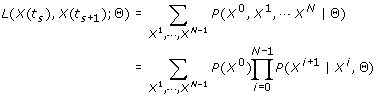(A.1)

For sufficiently large N, from the master equation Eq. (1.3), the conditional probability can be approximated by, where dt = (ts + 1- t s )/N and δ X', X is the Kronecker delta function.

We are interested in the gradient of the likelihood function instead of calculating the likelihood function explicitly. So we take the partial derivative of L(X(t s ), X(ts+1);Θ) w.r.t. the parameters,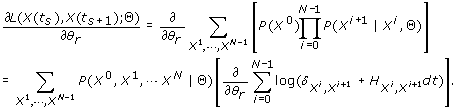(A.2)

Note that when N → ∞,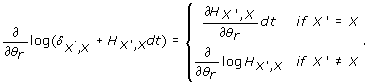Therefore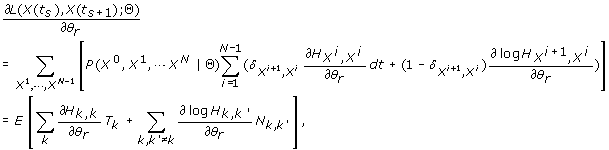(A.3)

where Tk is the time duration of the system at state k, and N k, k' is the number of transitions from state k to k' occurred during the interval. Both Tk and N k, k' are random variables, and can be viewed as the sufficient statistics of the model. E[·] represents the expectation of the random variables. For hazard functions of the form (1.5),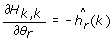and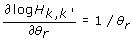, thus equation (2.1) and (2.4) follow.

The formula can also be derived by the time ordered product expansion described in  without resorting to time discretization, as shown below. This result (Eq. A.3) suggests that the gradient of the likelihood function can be calculated by estimating the expectation of the two sufficient statistics. Note that the formula is quite general and holds for any stochastic system obeying the master equation (Eq. 1.3).

### Derivation of the RJMCMC algorithm based on the time ordered product expansion of master equation

Representing the probability vector of system state as, the master equation (Eq. 1.3) can be written in a compact matrix form, using column vector notation (in this section),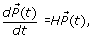where H is the time evolution matrix, of which the elements are uniquely determined by the stoichiometry matrix and the hazard functions.

The formal solution of master equation isThe probability of system evolving from a particular start state to an end state is given by the corresponding elements of the probability matrix. The time evolution matrix H is usually an infinite dimension matrix, for there are usually no upper bound for the species numbers.

The time ordered product expansion (TOPE) formula, which originates from quantum field theory, is useful to make series expansion of the matrix exponential. If we decompose the evolution matrix into two parts, H = H0 + H1, the TOPE formula giveswhere τ0 = t1, τ1 = t2 - t1 etc. A proper choice is to decompose H into diagonal and off-diagonal matrices H = Ĥ - D, i.e. H0 = - D and H1 = Ĥ. This leads to the TOPE formula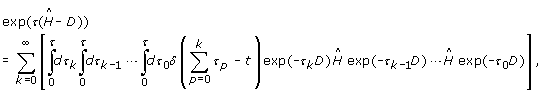where D represents the diagonal part (non-negative) and Ĥ is the off-diagonal part of the matrix. The terms inside the integral, conditioned on a given Markov jump process, is the likelihood (or probability density) of the process. In case of reaction systems, a process corresponds to a set of reaction events. Thus the k th order integration gives the total probability of all reaction events with k reactions. We note that the TOPE formula provides a possible way to estimate the matrix exponential (probability matrix) by Monte Carlo integration by randomly casting reaction events and summing up the likelihood.

In the fully observed case, the likelihood function is the product of the likelihood of each sub-interval,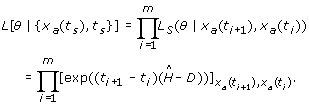The likelihood for each sub-interval can be denoted aswhere τ = ts+1- t s , and in the last step of the above equation we approximate the integration by a Monte-Carlo integral with π(Ξ({r i , t i })|θ r ) to be the likelihood of latent process Ξ({r i , t i }) (see Eq. 2.3) which is constrained by start/end observation (x a (ts+1) and x a (t s )) andin which n r is the number of reaction type r. V k is the multiplication of two parts: the first part arises from the simplex integration of the time variables, which can be viewed as the measure of integration space when we convert the integration to summation; the second part is a combinatorial factor resulting from the permutation invariance of the same reaction type in a given reaction path.

Recalling that in the RJMCMC algorithm, we generate samples with different number and type of reactions via the Metropolis-Hasting steps. The ratio between π(·)/V k of two samples gives the same acceptance probability as in Eq. (2.5).

Assuming all the reaction follows mass-action law, we can derive the gradient of the likelihood function using TOPE formula. We can write Ĥ and D matrix in terms of the component of each reaction type, i.e.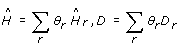. ThusWe define, which is the branching ratio for reaction r in state X'. Then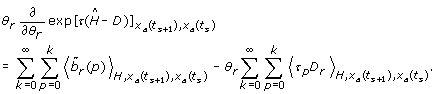Thus,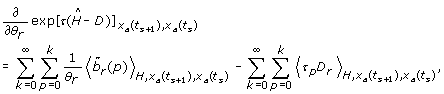which gives the gradient formula in Eq. (2.1), (2.4) and (A.3), since the average of a frequency gives the probability.

## Algorithm 1. Pseudo-code of RJMCMC algorithm for fully observed case

Input observations {n(t s )} and generate initial path for each interval using GLPK;

Calculate the null set {q' k } with the net-effect reaction matrix A;

for iter = 1: maxiteration

Randomly choose an elementary mode qk·;

for i = 1: number of time intervals

Randomly choose a move type p and update the reaction path in sub-interval [t i , t i+1 ) according to;

Calculate the number of each reaction type r m

if min(r m ) == 0, AP = 0; break

else

for j = 1: J (total number of reactions within the interval)

ifis negative for any a (1,2,...K),

AP = 0; break

else

Calculate the intermediate species number after the reaction: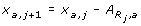endif

endfor

AP = min(1, AR ip );

endif

if AP > rand(0,1)

Accept the new path;

endif

endfor

endfor

## Algorithm 2. Pseudo-code of RJMCMC algorithm for partially observed case

Input observations {nΓ(t s )} and randomly specify state for the unobserved species, generate initial path for each interval with GLPK;

Calculate the null set {} using the partial reaction matrix A p ;

for iter = 1: maxiteration

for i = 1: number of time intervals

Randomly choose an elementary modeand a move type p; Update the reaction path in sub-interval (t i , ti+1) according to;

Calculate the number of each reaction type: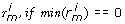, AP = 0; break

else

for j = 1: number of reactions within the ith interval

ifis negative for any species a AP = 0; break

else

Calculate the intermediate species number after the reaction:;

endif

endfor

endif

if xa, J + 1== x a (tt+1)else

Update the second interval via complementary move p';

Calculate the number of each reaction type: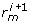if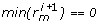, AP = 0; break

else

for j' = 1: number of reactions within the (i + 1)th interval

if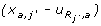is negative for any species a AP = 0; break

else

Calculate the intermediate species number after the reaction: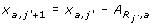;

endif

endfor

endif

Calculate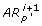for the new path and the acceptance probability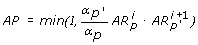;

endif

if AP > rand(0,1)

Accept the new path;

endif

endfor

endfor

## Algorithm 3: Stochastic gradient descent algorithm

Input: time-course dataOutput: set of inferred parameters {θ r }

1. Initialize the reaction path using GLPK and set initial values of rate parameters;

2. Sample the latent paths for the entire observation interval with reversible jump MCMC

-For fully observed case: sample latent paths for each interval s (0, m-1) using Algorithm 1;

-For partially observed case: sample latent paths for each paired intervals and separately for the first interval using Algorithm 2;

Calculate the gradient of each sample path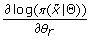according to Eq. (2.3) after burn in;

3. Calculate the gradient by averaging over sample paths;

4. Update parameter values by gradient descent step: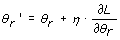for all r, where η is the step size;

5. If convergence condition (to be specified) is not satisfied, return to step 2.

## References

1. Kaern M, Elston TC, Blake WJ, Collins JJ: Stochasticity in gene expression: from theories to phenotypes. Nat Rev Genet. 2005, 6: 451-464. 10.1038/nrg1615

2. Elowitz MB, Levine AJ, Siggia ED, Swain PS: Stochastic gene expression in a single cell. Science. 2002, 297: 1183-1186. 10.1126/science.1070919

3. Swain PS, Elowitz MB, Siggia ED: Intrinsic and extrinsic contributions to stochasticity in gene expression. Proc Natl Acad Sci USA. 2002, 99: 12795-12800. 10.1073/pnas.162041399

4. Blake WJ, Kaern M, Cantor CR, Collins JJ: Noise in eukaryotic gene expression. Nature. 2003, 422: 633-637. 10.1038/nature01546

5. Pirone JR, Elston TC: Fluctuations in transcription factor binding can explain the graded and binary responses observed in inducible gene expression. J Theor Biol. 2004, 226: 111-121. 10.1016/j.jtbi.2003.08.008

6. Thattai M, van Oudenaarden A: Intrinsic noise in gene regulatory networks. Proc Natl Acad Sci USA. 2001, 98: 8614-8619. 10.1073/pnas.151588598

7. McAdams HH, Arkin A: Stochastic mechanisms in gene expression. Proc Natl Acad Sci USA. 1997, 94: 814-819. 10.1073/pnas.94.3.814

8. Ozbudak EM, Thattai M, Kurtser I, Grossman AD, van Oudenaarden A: Regulation of noise in the expression of a single gene. Nat Genet. 2002, 31: 69-73. 10.1038/ng869

9. Bar-Even A, Paulsson J, Maheshri N, Carmi M, O'Shea E, Pilpel Y, Barkai N: Noise in protein expression scales with natural protein abundance. Nat Genet. 2006, 38: 636-643. 10.1038/ng1807

10. Pedraza JM, van Oudenaarden A: Noise propagation in gene networks. Science. 2005, 307: 1965-1969. 10.1126/science.1109090

11. Choi PJ, Cai L, Frieda K, Xie XS: A stochastic single-molecule event triggers phenotype switching of a bacterial cell. Science. 2008, 322: 442-446. 10.1126/science.1161427

12. Arkin A, Ross J, McAdams HH: Stochastic kinetic analysis of developmental pathway bifurcation in phage lambda-infected Escherichia coli cells. Genetics. 1998, 149: 1633-1648.

13. Fraser HB, Hirsh AE, Giaever G, Kumm J, Eisen MB: Noise minimization in eukaryotic gene expression. PLoS Biol. 2004, 2: e137- 10.1371/journal.pbio.0020137

14. Becskei A, Serrano L: Engineering stability in gene networks by autoregulation. Nature. 2000, 405: 590-593. 10.1038/35014651

15. Rao CV, Wolf DM, Arkin AP: Control, exploitation and tolerance of intracellular noise. Nature. 2002, 420: 231-237. 10.1038/nature01258

16. Thattai M, van Oudenaarden A: Stochastic gene expression in fluctuating environments. Genetics. 2004, 167: 523-530. 10.1534/genetics.167.1.523

17. Schultz D, Ben Jacob E, Onuchic JN, Wolynes PG: Molecular level stochastic model for competence cycles in Bacillus subtilis. Proc Natl Acad Sci USA. 2007, 104: 17582-17587. 10.1073/pnas.0707965104

18. Beaumont HJE, Gallie J, Kost C, Ferguson GC, Rainey PB: Experimental evolution of bet hedging. Nature. 2009, 462: 90-93. 10.1038/nature08504

19. Lawrence ND, Girolami M, Rattray M, Sanguinetti G, : Learning and Inference in Computational Systems Biology. 2010, Cambridge, MA, The MIT Press,

20. Moles CG, Mendes P, Banga JR: Parameter estimation in biochemical pathways: a comparison of global optimization methods. Genome Res. 2003, 13: 2467-2474. 10.1101/gr.1262503

21. Bottou L: Stochastic learning. Lect Notes Artif Int. 2004, 3176: 146-168.

22. Kirkpatrick S, Gelatt C, Vecchi M: Optimization by Simulated Annealing. Science. 1983, 220: 671-680. 10.1126/science.220.4598.671

23. Janssens H, Hou S, Jaeger J, Kim A-R, Myasnikova E, Sharp D, Reinitz J: Quantitative and predictive model of transcriptional control of the Drosophila melanogaster even skipped gene. Nat Genet. 2006, 38: 1159-1165. 10.1038/ng1886

24. Chen WW, Schoeberl B, Jasper PJ, Niepel M, Nielsen UB, Lauffenburger DA, Sorger PK: Input-output behavior of ErbB signaling pathways as revealed by a mass action model trained against dynamic data. Mol Syst Biol. 2009, 5: 239-

25. Busch H, Camacho-Trullio D, Rogon Z, Breuhahn K, Angel P, Eils R, Szabowski A: Gene network dynamics controlling keratinocyte migration. Mol Syst Biol. 2008, 4: 199- 10.1038/msb.2008.36

26. Gillespie D: Exact stochastic simulation of coupled chemical reactions. J Phys Chem-Us. 1977, 81: 2340-2361. 10.1021/j100540a008.

27. Gillespie DT: Stochastic simulation of chemical kinetics. Annual review of physical chemistry. 2007, 58: 35-55. 10.1146/annurev.physchem.58.032806.104637

28. Li H, Cao Y, Petzold LR, Gillespie DT: Algorithms and software for stochastic simulation of biochemical reacting systems. Biotechnol Prog. 2008, 24: 56-61. 10.1021/bp070255h

29. Henderson DA, Boys RJ, Krishnan KJ, Lawless C, Wilkinson DJ: Bayesian Emulation and Calibration of a Stochastic Computer Model of Mitochondrial DNA Deletions in Substantia Nigra Neurons. J Am Stat Assoc. 2009, 104: 76-87. 10.1198/jasa.2009.0005.

30. Gillespie D: The chemical Langevin equation. J Chem Phys. 2000, 113: 297-306. 10.1063/1.481811.

31. Golightly A, Wilkinson DJ: Bayesian inference for stochastic kinetic models using a diffusion approximation. Biometrics. 2005, 61: 781-788. 10.1111/j.1541-0420.2005.00345.x

32. Golightly A, Wilkinson DJ: Bayesian sequential inference for stochastic kinetic biochemical network models. J Comput Biol. 2006, 13: 838-851. 10.1089/cmb.2006.13.838

33. Boys RJ, Wilkinson DJ, Kirkwood TBL: Bayesian inference for a discretely observed stochastic kinetic model. Stat Comput. 2008, 18: 125-135. 10.1007/s11222-007-9043-x.

34. Wilkinson DJ: Stochastic modelling for quantitative description of heterogeneous biological systems. Nat Rev Genet. 2009, 10: 122-133. 10.1038/nrg2509

35. Tian T, Xu S, Gao J, Burrage K: Simulated maximum likelihood method for estimating kinetic rates in gene expression. Bioinformatics. 2007, 23: 84-91. 10.1093/bioinformatics/btl552

36. Sisson SA, Fan Y, Tanaka MM: Sequential Monte Carlo without likelihoods. Proc Natl Acad Sci USA. 2007, 104: 1760-1765. 10.1073/pnas.0607208104

37. Toni T, Welch D, Strelkowa N, Ipsen A, Stumpf MPH: Approximate Bayesian computation scheme for parameter inference and model selection in dynamical systems. Journal of The Royal Society Interface. 2009, 6: 187-202. 10.1098/rsif.2008.0172.

38. Yosiphon G, Mjolsness E: Towards the inference of stochastic biochemical network and parameterized grammar models. Learning and Inference in Computational Systems Biology. Edited by: Lawrence ND, Girolami M, Rattray M, Sanguinetti G. 2009, 297-314. MIT Press,

39. Reinker S, Altman RM, Timmer J: Parameter estimation in stochastic biochemical reactions. Systems biology. 2006, 153: 168-178.

40. Henderson D, Boys R, Wilkinson D: Bayesian Calibration of a Stochastic Kinetic Computer Model Using Multiple Data Sources. Biometrics. 2009, 66: 249-56. 10.1111/j.1541-0420.2009.01245.x

41. Green P: Reversible jump Markov chain Monte Carlo computation and Bayesian model determination. Biometrika. 1995, 82: 711-732. 10.1093/biomet/82.4.711.

42. Brooks S, Giudici P, Roberts G: Efficient construction of reversible jump Markov chain Monte Carlo proposal distributions. J Roy Stat Soc B. 2003, 65: 3-39. 10.1111/1467-9868.03711.

43. Urbanczik R, Wagner C: An improved algorithm for stoichiometric network analysis: theory and applications. Bioinformatics. 2005, 21: 1203-1210. 10.1093/bioinformatics/bti127

44. von Kamp A, Schuster S: Metatool 5.0: fast and flexible elementary modes analysis. Bioinformatics. 2006, 22: 1930-1931. 10.1093/bioinformatics/btl267

45. Wright J, Wagner A: Exhaustive identification of steady state cycles in large stoichiometric networks. BMC systems biology. 2008, 2: 61- 10.1186/1752-0509-2-61

46. Edelstein-Keshet L: Mathematical Models in Biology. 2005, Society for Industrial and Applied Mathematics,

47. GLPK - GNU Linear Programming Toolkit. http://www.gnu.org/software/glpk/

48. GLPKMEX - a Matlab MEX interface for the GLPK library. http://sourceforge.net/projects/glpkmex/

49. Schuster S, Fell DA, Dandekar T: A general definition of metabolic pathways useful for systematic organization and analysis of complex metabolic networks. Nat Biotechnol. 2000, 18: 326-332. 10.1038/73786

50. Rabitz H: Systems Analysis at the Molecular Scale. Science. 1989, 246: 221-226. 10.1126/science.246.4927.221

51. Saltelli A, Ratto M, Tarantola S, Campolongo F: Sensitivity analysis for chemical models. Chem Rev. 2005, 105: 2811-2828. 10.1021/cr040659d

52. Rosenfeld N, Perkins TJ, Alon U, Elowitz MB, Swain PS: A fluctuation method to quantify in vivo fluorescence data. Biophys J. 2006, 91: 759-766. 10.1529/biophysj.105.073098

53. Rosenfeld N, Young JW, Alon U, Swain PS, Elowitz MB: Gene regulation at the single-cell level. Science. 2005, 307: 1962-1965. 10.1126/science.1106914

54. Clayton E, Doupe DP, Klein AM, Winton DJ, Simons BD, Jones PH: A single type of progenitor cell maintains normal epidermis. Nature. 2007, 446: 185-189. 10.1038/nature05574

55. Mjolsness E, Yosiphon G: Stochastic process semantics for dynamical grammars. Annals of Mathematics and Artificial Intelligence. 2006, 47: 329-395. 10.1007/s10472-006-9034-1.

## Acknowledgements

This work was partially supported by NSF grant DBI-0846218 to XX. SC was supported by NIH grants R01GM75309 and P50GM76516. EM was supported by grants NSF EF-0330786 and NIH R01GMO86883. We thank Jacob Biesinger, Qing Nie and Gui-Bo Ye for helpful discussions.

## Author information

Authors

### Corresponding author

Correspondence to Xiaohui Xie.

### Authors' contributions

The study was initially conceived by EM and YW, and later extended by SC and XX. YW implemented the algorithm and carried out most of the computational analysis. YW, SC and XX wrote the paper. All authors read and approved the final manuscript.

## Electronic supplementary material

### 12918_2010_488_MOESM1_ESM.DOCX

Additional file 1: Supplementary figures and tables. This file contains Supplementary Figure S1-S4, Table S1. (DOCX 977 KB)

## Authors’ original submitted files for images

Below are the links to the authors’ original submitted files for images.

## Rights and permissions

Reprints and Permissions

Wang, Y., Christley, S., Mjolsness, E. et al. Parameter inference for discretely observed stochastic kinetic models using stochastic gradient descent. BMC Syst Biol 4, 99 (2010). https://doi.org/10.1186/1752-0509-4-99

• Accepted:

• Published:

• DOI: https://doi.org/10.1186/1752-0509-4-99

### Keywords

• Reaction Path
• Flux Balance Analysis
• Acceptance Probability